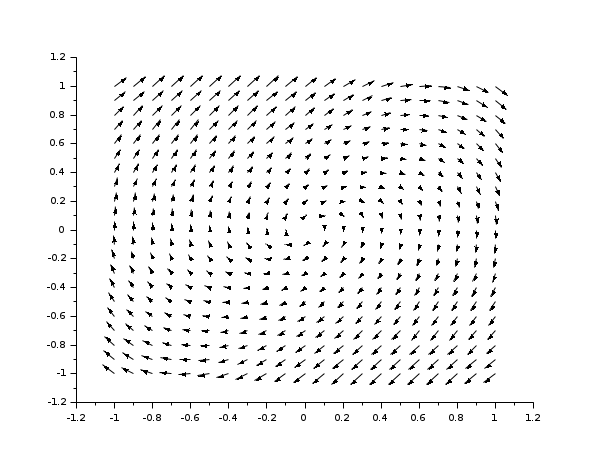Change language to:
English - Français - 日本語 - Português -

See the recommended documentation of this function

Справка Scilab >> Графики > 2d_plot > fchamp

# fchamp

direction field of a 2D first order ODE

### Syntax

```fchamp(f, t, xr, yr, [arfact, rect, strf])
fchamp(f, t, xr, yr, <opt_args>)```

### Arguments

f

an external (function or character string) or a list which describes the ODE.

-

It can be a function name `f`, where `f` is supposed to be a function of type `y=f(t, xy, [p1, ..., pn])`. `f` returns a column vector of size 2, `y`, which gives the value of the direction field `f` at point `xy=[x,y]` and at time `t`.

-

It can also be an object of type list, `list(f,P1, ..., Pn)` where `f` is a function of type `y=f(t, xy, p1, ..., pn)` and `Pi` gives the value of the parameter `pi`.

t

the selected time.

xr, yr

two row vectors of size `n1` and `n2` which define the grid on which the direction field is computed.

<opt_args>

This represents a sequence of statements `key1=value1, key2=value2`,... where `key1`, `key2`,... can be one of the following: `arfact`, `rect`, `strf` (see below).

arfact, rect, strf

Optional arguments, see `champ`.

### Description

`fchamp` is used to draw the direction field of a 2D first order ODE defined by the external function `f`.Note that if the ODE is autonomous, argument `t` is useless, but it must be given.

Enter the command `fchamp()` to see a demo.

### Examples

```deff("[xdot] = derpol(t,x)",..
["xd1 = x(2)";..
"xd2 = -x(1) + (1 - x(1)**2)*x(2)";..
"xdot = [ xd1 ; xd2 ]"])
xf= -1:0.1:1;
yf= -1:0.1:1;
fchamp(derpol,0,xf,yf)``````deff("[xdot] = derpol(t,x)",..
["xd1 = x(2)";..
"xd2 = -x(1) + (1 - x(1)**2)*x(2)";..
"xdot = [ xd1 ; xd2 ]"])
xf= -1:0.1:1;
yf= -1:0.1:1;
clf()
fchamp(derpol,0,xf,yf,1,[-2,-2,2,2],"011")```